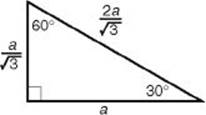﻿ ﻿SPECIAL RIGHT TRIANGLES - Triangles - PLANE GEOMETRY - SAT SUBJECT TEST MATH LEVEL 1

## PLANE GEOMETRY## CHAPTER 9 Triangles### SPECIAL RIGHT TRIANGLES

Let x be the length of each leg and let h be the length of the hypotenuse of an isosceles right triangle. By the Pythagorean theorem,Key Fact H7

In a 45-45-90 right triangle, the sides are x, x, and x.

If you are given the length of a leg, multiply it byto get the length of the hypotenuse.

If you are given the length of the hypotenuse, divide it byto get the length of each leg.EXAMPLE 5: To find the area of a square whose diagonal is 8, note that the diagonal divides the square into two isosceles right triangles. So.Let 2x be the length of each side of equilateralPQR, in which altitudehas been drawn. ThenPQS is a 30-60-90 right triangle, and its sides are x, 2x, and h. By the Pythagorean theorem,Key Fact H8

In a 30-60-90 right triangle, the sides are x, x, and 2x.
If you know the length of the shorter leg (x):

Multiply it byto get the length of the longer leg.

Multiply it by 2 to get the length of the hypotenuse.If you know the length of the longer leg (a):

Divide it byto get the length of the shorter leg.

Multiply the length of the shorter leg by 2 to get the length of the hypotenuse.If you know the length of the hypotenuse (h):

Divide it by 2 to get the length of the shorter leg.

Multiply the length of the shorter leg byto get the length of the longer leg.﻿